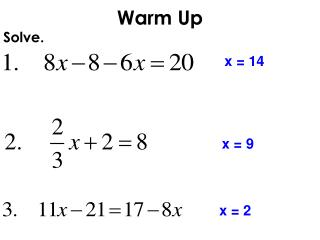# Warm Up - PowerPoint PPT PresentationDownload PresentationWarm Up

Warm Up
Download Presentation## Warm Up

- - - - - - - - - - - - - - - - - - - - - - - - - - - E N D - - - - - - - - - - - - - - - - - - - - - - - - - - -
##### Presentation Transcript

1. Warm Up x = 14 Solve. x = 9 x = 2

2. Symbols to Know

3. Name this angle 4 different ways.  C A 2  T 

4. Name the ways can you name 3? Name the ways can you name 4? Name the ways can you name MHT? M  A  3   4 T H

5. Name the angle 4 ways.

6. How do you name each red side? M A E U M T H Y F L I N

7. Angle Addition Postulate Why can’t you name any of the angles S? T R S P

8. Example 1 R T 1 P S

9. Linear Pair and Vertical Angles

10. Adjacent Angles Two angles are adjacent if they share a common vertex and side, but have no common interior points. SIDE BY SIDE…shoulder to shoulder. NO YES

11. Linear Pair Solve for x. x 62 Equation: ____ + ____ = 180 Two angles that are side-by-side and create a straight line (add up to 180). 118

12. Vertical Angles Solve for x. x 76 Equation: ______ = ______ 76 Their sides form two pairs of opposite rays (and the angles are equal to each other).

13. Solve for x. 50 Vertical Angles: Their sides form two pairs of opposite rays (and the angles are equal to each other).

14. Solve for x. 32° 1 _ x 3 96 Vertical Angles: Their sides form two pairs of opposite rays (and the angles are equal to each other).

15. Solve for x. 40° 100 Vertical Angles: Their sides form two pairs of opposite rays (and the angles are equal to each other).

16. Solve for x. (3x + 23)° (4x + 18)° 5 Vertical Angles: Their sides form two pairs of opposite rays (and the angles are equal to each other).

17. Supplementary Angles Solve for x if the following 2 angles are supplementary. x 82 Equation: ____ + ____ = 180 98 Two angles add up to 180.

18. Solve for x. 23 Supplementary Angles: Two angles add up to 180.

19. 13 and 14 are supplementary angles m13 = 47. Find m14. 133 Supplementary Angles: Two angles add up to 180.

20. Complementary Angles Solve for x if the following 2 angles are complementary. x 76 Equation: ____ + ____ = 90 14 Two angles add up to 90.

21. Solve for x. 2x + 23 x + 13 18 Complementary Angles: Two angles add up to 90.

22. Review 1 5 2 4 3 no Are angles 4 and 5 supplementary angles? no Are angles 2 and 3 complementary angles? Are angles 4 and 3 supplementary angles? yes Are angles 2 and 1 complementary angles? yes